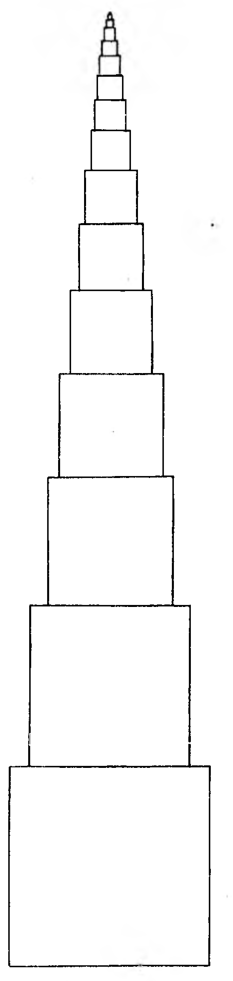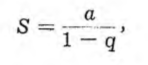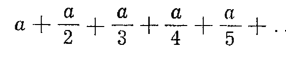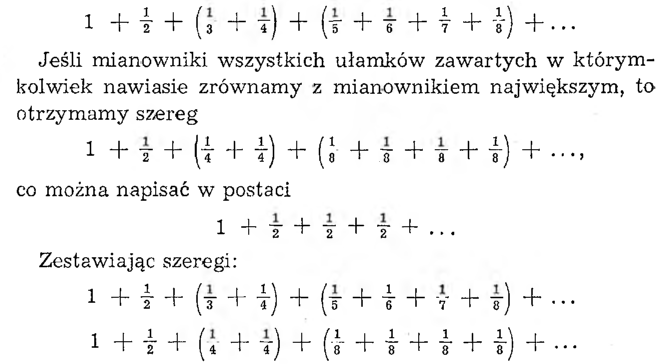# Exhibition emblemAn exhibition was designed. The management has announced a competition for the emblem of the exhibition.

Among, of the submitted projects, there were two ideas on quite similar topics.
He proposed one of these projects, that the exhibition's emblem would be a pyramid of stacked cubes: on the great cube with the edge a = 25 m a cube with an edge o 20% smaller, on it a new cube with the edge of Fr. 20% smaller than the edge of the previous cube, and so on.
The second design placed a cube with the edge a = in the base of the pyramid 25 m, on it was supposed to be a cube with an edge ½ a, then, in turn, cubes with edges 1/3 a, 1/4 a and so on.

Which of these cube pyramids will be higher??

It turns out, that the first tower will have 125 meters high. In fact, you need to calculate the sum of the geometric series

25 + 25 • 4/5 + 25 • (4/5)² + 25 • (4/5)³+ . . .

As you know, such sum is expressed by the formulawhere a is the first term of the series, a q — jego iloraz. In this case, we have a - 25, q = 4/5, so S = 125.

To calculate the height of the second tower, the sum of this series must be found:Such a series is called a harmonic series. Well, this is a divergent series, for the sum of his words taken in sufficient quantity, it can exceed any size, predetermined number.

This is easy to see from somewhat original reasoning. The words of this series can be combined into such groups:we find, that each parenthesis in the first series contains a word greater than the corresponding parenthesis in the second series. But the second row is divergent, hence, the first row is also divergent.

So we see, that the tower of this truly impressive design would have to be pulled up forever, but we would have gotten to that height along the way, at which the centrifugal force due to the rotation of the Earth about the axis would be greater than the force of attraction of the Earth.